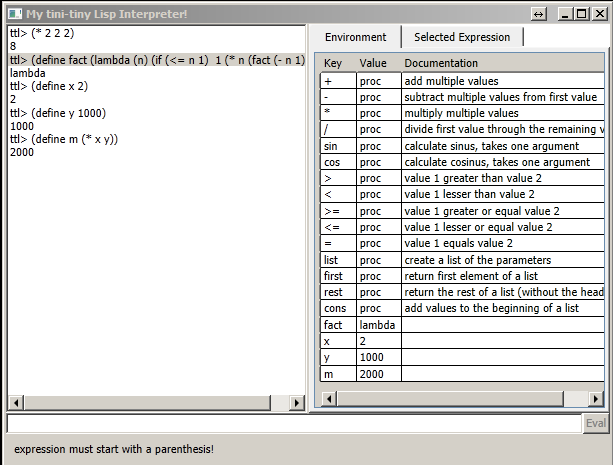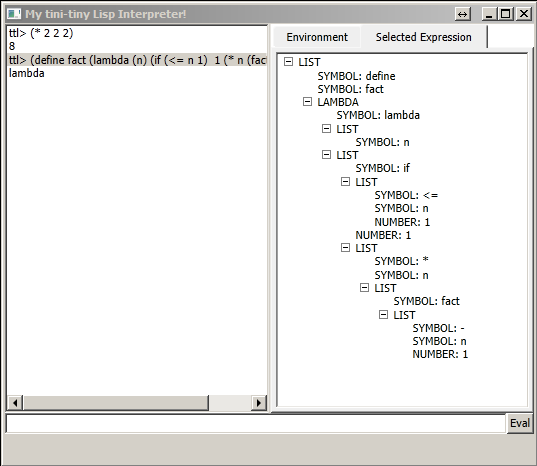# A Lisp Interpreter

## Supported Operators

• quote, if, lambda
• Arithmetic operators: +, *, -, /
• List operations: first, rest, cons, list
• Comparisons: >, >=, <, <=, =
minimaler Funktionsumfang f³r Lisp

## User interface

The main part of the user interface consists of a textbox for user inputs and a listbox for displaying in/out statements. In additon, the user interface shows the elements of the current environment and a tree like representation of the selected expression.## Sample in/out

```	ttl>(define x 2)
2
ttl>(* x 5)
10
ttl>(set x (+ x x))
4
ttl>(define square(lambda (x) (* x x)))
lambda
ttl>(square x)
16
ttl>(define compose (lambda (f g) (lambda (x) (f (g x)))))
lambda
ttl>((compose square square) 2)
16
ttl>(define  fact (lambda (n) (if (<= n 1) 1 (* n (fact (- n 1))))))
lambda
ttl>(fact 10)
3628800
ttl>
```